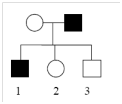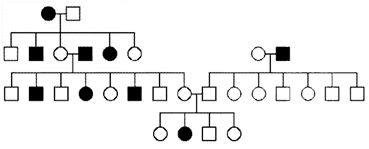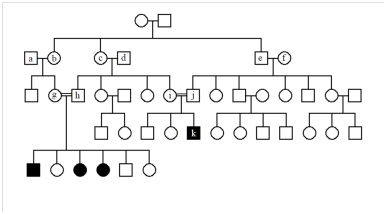# 5.E: Pedigrees and Populations (Exercises)

$$\newcommand{\vecs}{\overset { \rightharpoonup} {\mathbf{#1}} }$$ $$\newcommand{\vecd}{\overset{-\!-\!\rightharpoonup}{\vphantom{a}\smash {#1}}}$$$$\newcommand{\id}{\mathrm{id}}$$ $$\newcommand{\Span}{\mathrm{span}}$$ $$\newcommand{\kernel}{\mathrm{null}\,}$$ $$\newcommand{\range}{\mathrm{range}\,}$$ $$\newcommand{\RealPart}{\mathrm{Re}}$$ $$\newcommand{\ImaginaryPart}{\mathrm{Im}}$$ $$\newcommand{\Argument}{\mathrm{Arg}}$$ $$\newcommand{\norm}{\| #1 \|}$$ $$\newcommand{\inner}{\langle #1, #2 \rangle}$$ $$\newcommand{\Span}{\mathrm{span}}$$ $$\newcommand{\id}{\mathrm{id}}$$ $$\newcommand{\Span}{\mathrm{span}}$$ $$\newcommand{\kernel}{\mathrm{null}\,}$$ $$\newcommand{\range}{\mathrm{range}\,}$$ $$\newcommand{\RealPart}{\mathrm{Re}}$$ $$\newcommand{\ImaginaryPart}{\mathrm{Im}}$$ $$\newcommand{\Argument}{\mathrm{Arg}}$$ $$\newcommand{\norm}{\| #1 \|}$$ $$\newcommand{\inner}{\langle #1, #2 \rangle}$$ $$\newcommand{\Span}{\mathrm{span}}$$$$\newcommand{\AA}{\unicode[.8,0]{x212B}}$$

These are homework exercises to accompany Nickle and Barrette-Ng's "Online Open Genetics" TextMap. Genetics is the scientific study of heredity and the variation of inherited characteristics. It includes the study of genes, themselves, how they function, interact, and produce the visible and measurable characteristics we see in individuals and populations of species as they change from one generation to the next, over time, and in different environments.

## Study Questions

1. What are some of the modes of inheritance that are consistent with this pedigree?2. In this pedigree in question 1, the mode of inheritance cannot be determined unamibguously.What are some examples of data (e.g. from other generations) that, if added to the pedigree would help determine the mode of inheritance?

3. For each of the following pedigrees, name the most likely mode of inheritance (AR=autosomal recessive, AD=autosomal dominant, XR=X-linked recessive, XD=X-linked dominant).(These pedigrees were obtained from various external sources).

a)b)c)d)4. The following pedigree represents a rare, autosomal recessive disease. What are the genotypes of the individuals who are indicated by letters?5. If individual #1 in the following pedigree is a heterozygote for a rare, AR disease, what is the probability that individual #7 will be affected by the disease? Assume that #2 and the spouses of #3 and #4 are not carriers.6. You are studying a population in which the frequency of individuals with a recessive homozygous genotype is 1%. Assuming the population is in Hardy-Weinberg equilibrium, calculate:

a) The frequency of the recessive allele.

b) The frequency of dominant allele.

c) The frequency of the heterozygous phenotype.

d) The frequency of the homozygous dominant phenotype.

7. Determine whether the following population is in Hardy-Weinberg equilibrium.

 genotype number of individuals AA Aa aa 432 676 92

8. Out of 1200 individuals examined, 432 are homozygous dominant (AA)for a particular gene. What numbers of individuals of the other two genotypic classes (Aa, aa) would be expected if the population is in Hardy-Weinberg equilibrium?

9. Propose an explanation for the deviation between the genotypic frequencies calculated in question 8 and those observed in the table in question 7.

5.1 The pedigree could show an AD, AR or XR mode of inheritance. It is most likely AD. It could be AR if the mother was a carrier, and the father was a homozygote. It could be XR if the mother was a carrier, and the father was a hemizygote. It cannot be XD, since the daughter (#2) would have necessarily inherited the disease allele on the X chromosome she received from her father.

5.2 There are many possible answers. Here are some possibilities: if neither of the parents of the father were affected (i.e. the paternal grandparents of children 1, 2, 3), then the disease could not be dominant. If only the paternal grandfather was affected, then the disease could only be X-linked recessive if the paternal grandmother was a heterozygote (which would be unlikely given that this is a rare disease allele).

5.3 a) The mode of inheritance is most likely AD, since every affected individual has an affected parent, and the disease is inherited even in four different matings to unrelated, unaffected individuals. It is very unlikely that it is XD or XR, in part because affected father had an affected son.

b) The mode of inheritance cannot be AD or XD, because affected individuals must have an affected parent when a disease allele is dominant. Neither can it be XR, because there is an affected daughter of a normal father. Therefore, it must be AR, and this is consistent with the pedigree.

c) The mode of inheritance cannot be AD or XD, because, again, there are affected individuals with unaffected parents. It is not XR, because there are unaffected sons of an affected mother. It is therefore likely AR, but note that the recessive alleles for this condition appear to be relatively common in the population (note that two of the marriages were to unrelated, affected individuals).

d) The mode of inheritance cannot be AD or XD, because, again, there are affected individuals with unaffected parents. It could be either XR or AR, but because all of the affected individuals are male, and no affected males pass the disease to their sons, it is likely XR.

5.4 If a represents the disease allele, individuals a, d, f (who all married into this unusual family) are AA, while b, c, e, g, h, i, j are all Aa, and k is aa.

5.5 There is a ½ chance that an offspring of any mating Aa x AA will be a carrier (Aa). So, there is a ½ chance that #3 will be Aa, and likewise for #4. If #3 is a carrier, there is again a ½ chance that #5 will be a carrier, and likewise for #6. If #5 and #6 are both Aa, then there is a ¼ chance that this monohybrid cross will result in #7 having the genotype aa, and therefore being affected by the disease. Thus, the joint probability is 1/2 x 1/2 x 1/2 x 1/2 x 1/4 =1/64.

5.6 a) q = -0.01. = 0.1

b) 1-q = p; 1-0.1 = 0.9

c) 2pq = 2(0.1)(0.9) = 0.18

d) p2 = 0.81

5.7 First, calculate allele frequencies:

$\mathrm{p = \dfrac{2(AA) + (Aa)}{total\: number\: of\: alleles\: scored} = \dfrac{2(432) + 676}{2(432+676+92)} = 0.6417}$

$\mathrm{q = \dfrac{2(aa) + (Aa)}{total\: number\: of\: alleles\: scored} = \dfrac{2(92) + 676}{2(432+676+92)} = 0.3583}$

Next, given these observed allele frequencies, calculate the genotypic frequencies that would be expected if the population was in Hardy-Weinberg equilibrium.

$\mathrm{p^2 = 0.6417^2 = 0.4118}$

$\mathrm{2pq = 2(0.6417)(0.3583) = 0.4598}$

$\mathrm{q^2 = 0.3583^2 = 0.1284}$

Finally, given these expected frequencies of each class, calculate the expected numbers of each in your sample of 1200 individuals, and compare these to your actual observations.

 expected observed (reported in the original question) AA 0.4118 × 1200 = 494 Aa 0.4598 × 1200 = 552 aa 0.1284 × 1200 = 154 432 676 92

The population does not appear to be at Hardy-Weinberg equilibrium, since the observed genotypic frequencies do not match the expectations. Of course, you could do a chi-square test to determine how significant the discrepancy is between observed and expected.

5.8 If in this theoretical question, the frequency of genotype of AA is set at 432/1200 and we are asked what frequencies of the other classes would fit a Hardy-Weinberg equilibrium. So, given that p2 = 432/1200, then p=0.6, and q=0.4. Given these allele frequencies and a sample size of 1200 individuals, then there should be 576 Aa individuals (2pq × 1200 = 2(0.6)(0.4) × 1200=576) and 192 aa individuals (q2 × 1200 = 0.42 × 1200 = 192), if the population was at Hardy-Weinberg equilibrium with 432 AA individuals.

5.9 The actual population appear to have more heterozygotes and few recessive homozygotes than would be expected for Hardy-Weinberg equilibrium. There are many possible reasons that a population may not be in equilibrium (see Table 5.1). In this case, there is possibly some selection against homozygous recessive genotypes, in favour of heterozygotes in particular. Perhaps the heterozygotes have some selective advantage that increases their fitness.

It is also worth noting the discrepancies between the allele frequencies calculated in 5.8 and 5.7. In question 5.7, we calculated the frequencies directly from the genotypes (this is the most accurate method, and does not require the population to be in equilibrium). In 5.8, we essentially estimated the frequency base on one of the phenotypic classes. The discrepancy between these calculations shows the limitations of using phenotypes to estimate allele frequencies, when a population is not in equilibrium.

This page titled 5.E: Pedigrees and Populations (Exercises) is shared under a CC BY-SA 3.0 license and was authored, remixed, and/or curated by Todd Nickle and Isabelle Barrette-Ng via source content that was edited to the style and standards of the LibreTexts platform; a detailed edit history is available upon request.